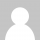# 震惊小伙伴的单行代码●Groovy篇

## 1、让列表中的每个元素都乘以2

```(1..10)*.multiply(2)
```

## 2、求列表中的所有元素之和

```(1..1000).sum()
```

## 3、判断一个字符串中是否存在某些词

```def wordList = ['groovy', 'akka', 'grails framework', 'spock', 'typesafe']
def tweet = 'This is an example tweet talking about groovy and spock.'
wordList.any { word -> tweet.contains(word) }
```

## 4、读取文件

```def fileText = new File('data.txt').text
```

## 5、祝你生日快乐！

```(1..4).each { println 'Happy Birthday ' + ((it == 3) ? 'dear Arturo' : 'to You') }
```

## 6. 过滤列表中的数值

```def (passed, failed) = [49, 58, 76, 82, 88, 90].split{ it > 60 }
```

## 7. 获取XML web service数据并分析

```def results = new XmlSlurper().parse('http://search.twitter.com/search.atom?&q=groovy')
```

## 8. 找到列表中最小或最大的一个数字

```[14, 35, -7, 46, 98].min()
[14, 35, -7, 46, 98].max()
```

## 9. 并行处理

```import groovyx.gpars.*
GParsPool.withPool { def result = dataList.collectParallel { processItem(it) } }
```

Gpars提供了直观安全的在Groovy里执行并行任务。

## 10. “Sieve of Eratosthenes”算法

```def t = 2..100
(2..Math.sqrt(t.last())).each { n -> t -= ((2*n)..(t.last())).step(n) }
println t```

### One Response to 震惊小伙伴的单行代码●Groovy篇

1.shopee虾皮代入驻开店亚马逊 says:

希望能学有所成。